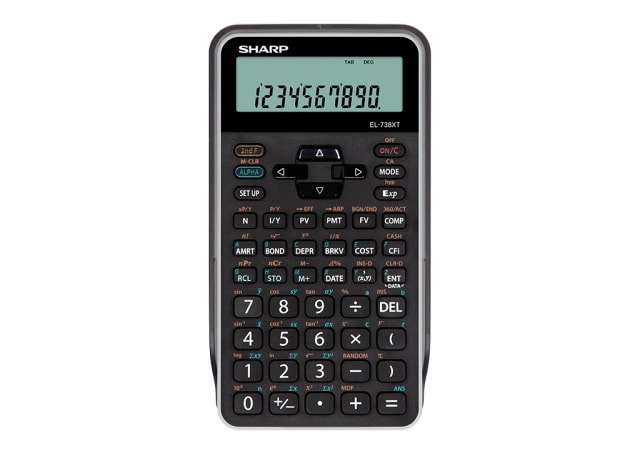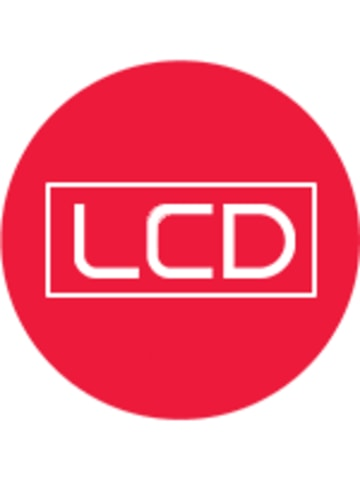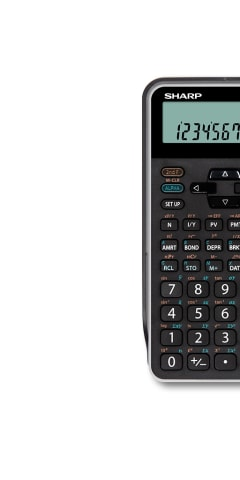EL738XTB

# Financial Calculator With Scientific Functions

• Large 2 line LCD display, with 3-digit punctuation.
• Acrylic Panel to protect LCD.
• Advanced finance and business functions including Compound Interest, Amortisation, Cash Flow Analysis, Depreciation and Break Even.
• 1-variable & 2-variable Statistics calculations.
• Handy Scientific functions.
• 12 memories.
• Easy to use Cost/Sell/Margin function.
• Multi-line Playback.
• Day/Date function for calculating days between dates, as well as new dates.
• Bond calculation
POA## Scientific Calculator## LCD Display## Battery Operated## Protective Hard Cover• A scientific calculator designed for usage in science, engineering, and mathematics. Great for school/university students or engineers.
• 2-line display showing equation entered on top line and answer on the bottom line allowing users to check both the equation and answer at the same time. User can amend the equation if needed.
• Lets you recall the steps you have made (up to capacity of memory). This feature is very useful for comparing formulas and recalculating equations.
• Hard cover to protect calculator from scratches and for easy storage. Great for students to store their calculators in their school bags.
• To calculate cost, selling price and margin/markup price of any item or good.
• A financial function used in order to solve bond prices or yields to maturity with accrued interest. Great for student/university students or bankers/financial planners.
• For easier reading of long, multi-digit numbers, "," or " , " is inserted for each group of three digits e.g. 10 thousand will be displayed as 10,000.
• Used for calculating between dates and new dates.
• Allows you to enter numbers as fractions.
• Finance and business functions can be calculated including Compound Interest, Amortisation, Cash Flow Analysis, Depreciation and Break Even.
• To use the calculator for 1-2 variable statistic calculations. Great for students or engineers.
Calculator Types
Scientific Calculators
Display
2 Line LCD
Dimensions (WxHxD)
77 x 150 x 12 millimetre
Power Source
Battery CR2032
Memory
12
Chain Calculation
Yes
Finance functions
Yes
Statistics mode
Yes
Hard Cover
Yes, slide-on